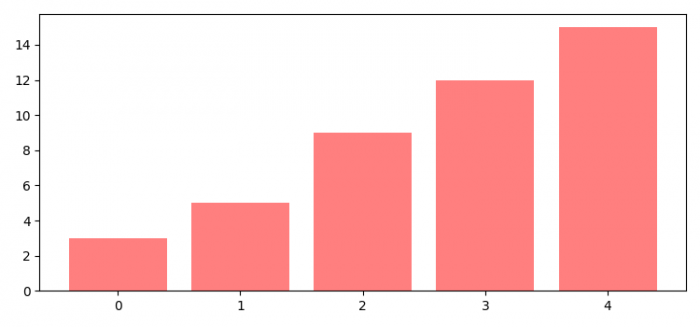# How to sort bars in a bar plot in ascending order (Matplotlib)?

To sort bars in a bar plot in ascending order, we can take the following steps −

• Set the figure size and adjust the padding between and around the subplots.

• Make a list of data for bar plots.

• Create a bar plot using bar() method, with sorted data.

• To display the figure, use show() method.

## Example

from matplotlib import pyplot as plt

plt.rcParams["figure.figsize"] = [7.50, 3.50]
plt.rcParams["figure.autolayout"] = True

data = [3, 5, 9, 15, 12]

plt.bar(range(len(data)), sorted(data), color='red', alpha=0.5)

plt.show()

## Output